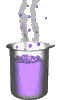Science class online
Chemistry and physics lessons

# Mechanics

Free online mechanics lesson for elementary school,  middle school and high school.Science class Chemistry Electricity Optics Mechanics Mechanics lessons Gravity - The solar system - motions of celestial bodies - Physical interactions - Effects of gravity - Weight of an object - Difference between weight and mass - Relationship between weight and mass  Energy - Energy basics - Potential energy - Kinetic energy - Mechanical energy - Translational Kinetic Energy - kinetic energy mass and speed

 _____________________________________________________     _____________________________________________________

## Gravity

### Weight of an object

 1) What is weight ? For an object on Earth we can define weight as follows: Weigth is the gravitational action exerted by Earh upon this object. The weight can also be defined for objects on other celestial bodies. For instance, on the Moon the weight is the gravitational action exerted by the Moon upon an object. ______________________________________ ______________________________________ 2) Weight properties - Axis and direction: If an object is dropped it falls down along a vertical axis. The weight has the same properties: it is exerted along a vertical axis oriented downwardly. At the Earth's scale, the weight is exerted following the direction of terrestrial radius, toward its center. - The weight value: The weight value is denoted W. It is expressed in newton ( symbol: N ) We can measure weight using a dynamometer. ______________________________________ ______________________________________
____________________________________

____________________________________